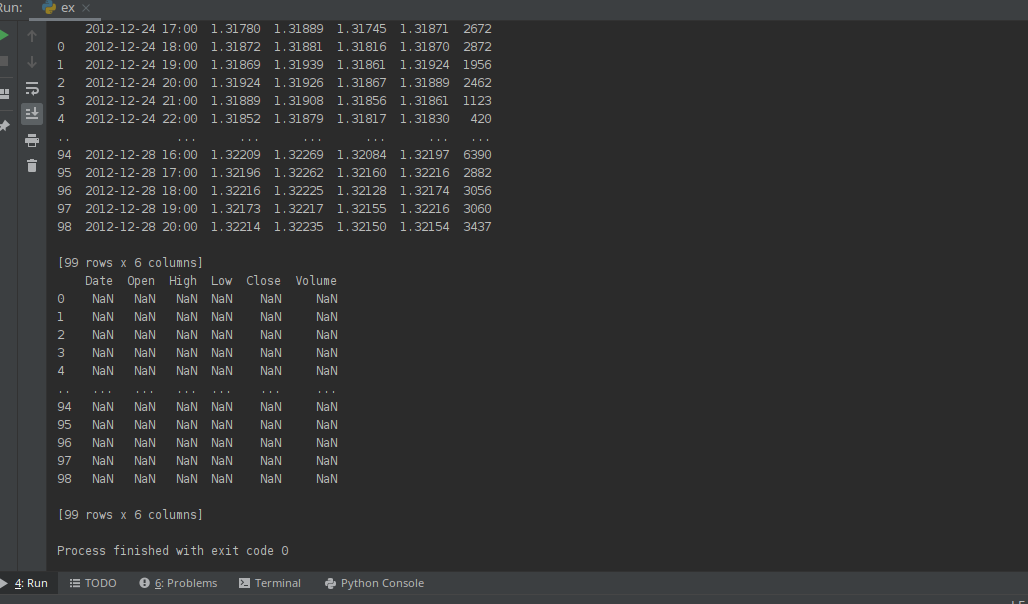Python Forum

Full Version: Outputs "NaN" after "DataFrame columns" function?
You're currently viewing a stripped down version of our content. View the full version with proper formatting.
import numpy as np
import pandas as pd
import matplotlib.pyplot as plt
from scipy.signal import argrelextrema

print(data)

data = pd.DataFrame(data=data, columns=["Date", "Open", "High", "Low", "Close", "Volume"])

print(data)
and output is:I'm trying some strategies and calculations on prices. Previously I was working on windows (I was using excel for the python file) then I installed manjaro and encountered such problem with the csv file.
NaN is missing data or None (can be used interchangeably).
(Jan-23-2021, 10:53 PM)Larz60+ Wrote: [ -> ]NaN is missing data or None (can be used interchangeably).

Yes I know, but why ? :)As you can see in the picture, when there is data after the first "read_csv", NaN returns after naming the columns.
First change line 10 to:
df = pd.DataFrame(data=data, columns=["Date", "Open", "High", "Low", "Close", "Volume"])
you were overwriting 'data'.

Then, to isolate the data, please change line 8 to:
print(f"\nCSV data:\n{data}")

and line 12 to:
print(f"\ndataframe:\n{df}")

Show results again
(Jan-24-2021, 06:35 PM)Larz60+ Wrote: [ -> ]First change line 10 to:
df = pd.DataFrame(data=data, columns=["Date", "Open", "High", "Low", "Close", "Volume"])
you were overwriting 'data'.

Then, to isolate the data, please change line 8 to:
print(f"\nCSV data:\n{data}")

and line 12 to:
print(f"\ndataframe:\n{df}")

Show results again

import numpy as np
import pandas as pd
import matplotlib.pyplot as plt
from scipy.signal import argrelextrema

print(f"\nCSV data:\n{data}")

df = pd.DataFrame(data=data, columns=["Date", "Open", "High", "Low", "Close", "Volume"])

print(f"\ndataframe:\n{df}")
I did, but the result is the same as in the picture
The new output should contain the two print statements, which will isolate the output to the different sections of software. That was what I was interested in seeing.

If you don't see the print statements, something else is happening here.
Looking at the screenshot, note that initial data dataframe does not have these columns. Confirmed by 99 rows x6 columns (index is 0 to 98)
index 0 is 2012-12-14 18:00, and the column names are values for 2012-12-24 17:00

I guess your csv file has no header and you need to specify columns when reading it.
Can you show your file (e.g. top 10 rows of it?)

or try
data = pd.read_csv("Data/EURUSD60.csv", delimiter="\t", names=["Date", "Open", "High", "Low", "Close", "Volume"])
(Jan-26-2021, 07:19 PM)buran Wrote: [ -> ]Looking at the screenshot, note that initial data dataframe does not have these columns. Confirmed by 99 rows x6 columns (index is 0 to 98)
index 0 is 2012-12-14 18:00, and the column names are values for 2012-12-24 17:00

I guess your csv file has no header and you need to specify columns when reading it.
Can you show your file (e.g. top 10 rows of it?)

or try
data = pd.read_csv("Data/EURUSD60.csv", delimiter="\t", names=["Date", "Open", "High", "Low", "Close", "Volume"])

Yes, there is no title in the csv-file, as you said, I added a header(name=[..]) in "read_csv" and it's okay.
Thanks!